## 6 Activities using Havi Elements to teach Circle area, circumference and PiIf a Mathematician opens a bakery, what would he name it?
“Drumroll”
“House of Pi”
Or “Eatable Shapes”?

Yes yes, we know that we need to work on our sense of humour. So let’s jump start on the six activities which you can do using Havi Elements to understand the concepts of circle, area and circumference of the circle, and the Pi.

What you need?

Elements:
Power – 1
Motor – 2

Accessories:
Car plate
Wheels – 2
Roller – 1
Geared motors – 2

Craft material:
White sheets
Watercolor
Sketch pen/ marker pen
Double-sided tape

Activity 1

You have the wheel. And one ant is walking on the surface of the wheel. How much distance would it cover when it completes one round?

Do you have a scale? And can you measure the distance using the scale?

Not directly, because you can not wrap the scale on the surface of the wheel.

One possible way is to take the wheel grip out, cut it, and spread it to length somehow. Measure the length and the Ant would have travelled this much.

BUT, BUT DON’T DO THAT. DON’T CUT THE GRIP.

We have a better way.

Let’s take a piece of thread. Wrap it around the wheel. Start and end the wrap at the same spot on the wheel. And now measure this length of the string using a scale.

That’s what the ant would have travelled on the surface.

What’s the value? That value is also called the Circumference or the Perimeter of the circle.
Let’s call this value c. What’s your c?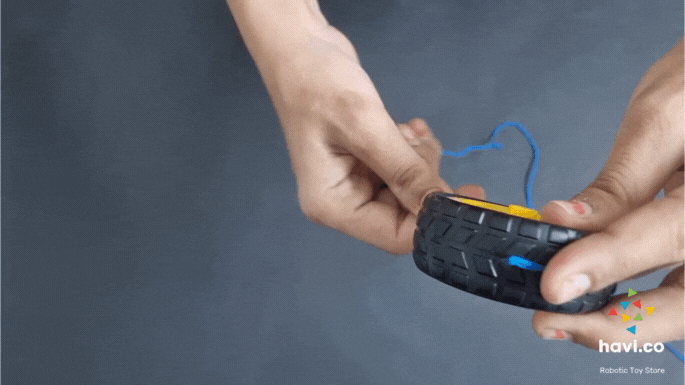Activity 2

Take a paper sheet and watercolor.

Make a darker dot on the wheel using the color.

Now, roll the wheel on the paper so that the wheel marks two dots. Measure the distance between the two dots.

Is it the same as the c we have found in activity 1?

It must be the same or very nearby.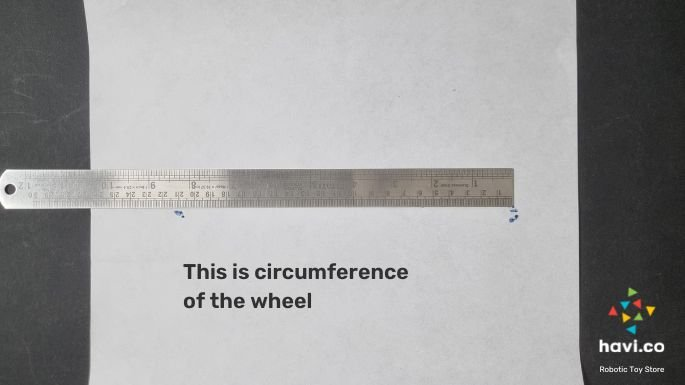Activity 3

Now let’s make a car using Havi Elements.

And place a color dot on each of the wheels and let the car run on a sheet. Each of the wheels will make dots on the sheet. And again, the distance between the two dots is equal to the circumference we have found already.

So now we know that the circumference of a circle is the linear distance around it. And we have found the circumference using 3 activities already.Activity 4

Let’s draw a circle using the car made using Havi elements.

Make Havi elements car but keep only one motor On. Detach one of the geared motors with the motor element. Hence the car will be powered by only one geared motor.

Mark a dot on the sheet using the marker pen and place the car on the sheet in a way so the Off wheel stays on the mark you have made.

The car will rotate in a circle with the off-wheel as the center point.

If you place a sketch pen or marker pen with the car, it will draw a circle.

This circle is the collection of all the smaller dots the pen is made of. And all these dots are at an equal distance from the center point.

So the circle is a collection of all the dots at an equal distance from the center point.
And that distance is called the radius of the circle.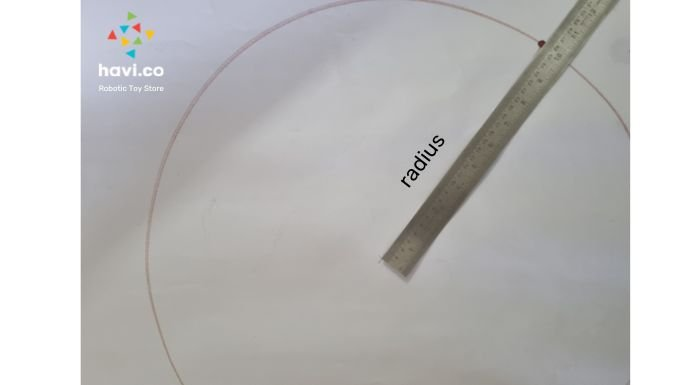So here measure the distance of the circle line from the center point (the mark you have made on the sheet).

Measure the distance from one point of the circle to the opposite point, keeping the scale so that it passes through the center point.

This distance is your diameter. Call it d.Activity 5

Measure the diameter of the wheel using a scale.

You already know the circumference, as we have found in the activity 1, 2, and 3.

Divide the circumference by the diameter.

How do I know? Before I tell you that, take any other round shape and find its circumference and diameter. Divide the circumference by the diameter.

3.14

Yes, for a circle of any size, if you divide the circumference by the diameter, the answer will be 3.14.

This 3.14 is a constant with infinite digits after 14 and is called Pi.

Pi = Circumference/ diameter.
Circumference = diameter * Pi

Hurray… you no more need strings and colors to measure the circumference of the circle. Simply multiply the diameter with Pi and you will get the circumference.

Activity 6

Make 16 equal parts of the circle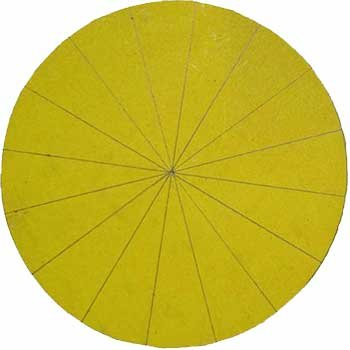Number each of the parts.Cut the circle into these 16 parts.

Put the part number 1 upright and 2nd near to it, but upside down. Place all parts this way and they will form a Parallelogram.Assume a rectangle out of this parallelogram.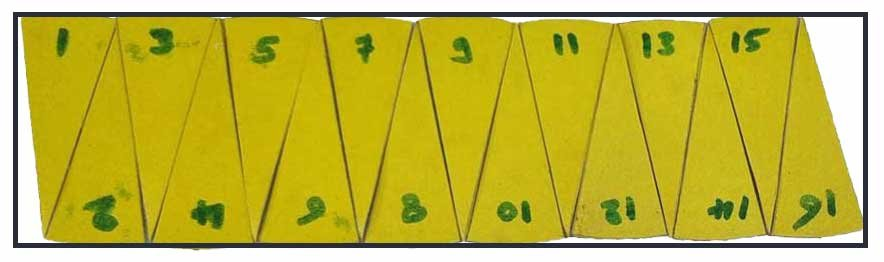Measure the width and height of the rectangle.

The height of this would be the radius of the circle. Check that out!

The width would be half of the circumference of the circle.

What is the area of a rectangle? Multiply the width and the height, that’s the “area” of your circle.

What is the area?

Any physical object occupies some space. That space is the area of that object. Even a circle has its area.

One more time, have a look at the width and height.
And width = Pi * radius.

Yes, width of this rectangle, formed out of 16 parts of the circle will always be the multiplication of Pi and the radius. Try doing this with 3 different circles of 3 different radiuses.

So now area = height * width
That means

Yuhuuuu…. We have one more easy formula, this time to calculate the area of the circle.

That’s it.

Try it yourself.

### Havi Camp

[time_change], 6 PM IST, on zoom call.

A class of Technology and future skills for age 5 to 15.

Get an invite

[intl_tel* intl_tel-866 class:single_contct1 initialCountry:in]

We never spam, never sell or share your data and never track and target you with ads.

0
0